# Mathematical problems for self assessment

###### INTEGRATION I

1. Evaluate the indefinite integrals:

(i)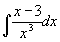(ii)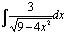[Ans. (i)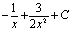(ii)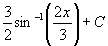]

2. Evaluate the indefinite integrals:

(i)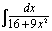(ii)[Ans. (i)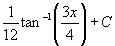(ii)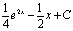]

3. Calculate the area under the curve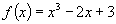between x = 1 and x = 2.

[Ans. 15/4]

4. Calculate the area bounded by the curves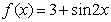and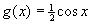over the region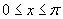.

[Ans. 3π ]

5. Evaluate the definite integrals:

(i)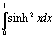(ii)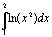(Hint: Use integration by parts)

[Ans. (i)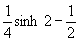(ii)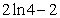]

6. Using integration by parts evaluate the indefinite integral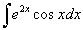[Ans.]

7. Using integration by parts evaluate the definite integral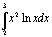[Ans.]

8. Evaluate the improper integral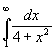[Ans.]

9. Using integration by parts find a reduction formula for the improper integral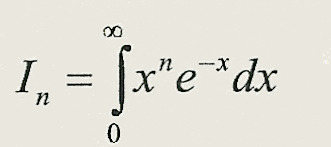and hence evaluate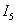[Ans.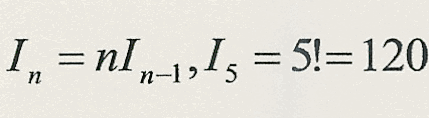].